# Chemical Engineering - Fluid Mechanics

6.

The energy equation,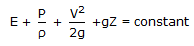(E = internal energy/mass), is applicable to

 A. perfect gases only. B. isothermal flow of gases. C. adiabatic unsteady flow of gases. D. all compressible fluids.

Explanation:

No answer description available for this question. Let us discuss.

7.

What is the ratio of fluid carrying capacity of two pipes having diameters d1 and d2 respectively ?

 A.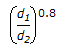B.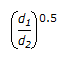C.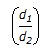D.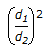Explanation:

No answer description available for this question. Let us discuss.

8.

A differential pressure cell is used for

 A. measuring small pressure difference in gases. B. measuring small pressure difference in liquids. C. remote recording of pressure difference. D. measuring the difference of the impact & the static pressure.

Explanation:

No answer description available for this question. Let us discuss.

9.

If more than two branches of pipes are to be connected at the same point, then use a/an

 A. elbow B. union C. tee D. none of these.

Explanation:

No answer description available for this question. Let us discuss.

10.

In case of a centrifugal pump, the ratio of total delivered pressure to pressure developed with the impeller is called the __________ efficiency.

 A. manometric B. mechanical C. volumetric D. overall